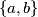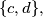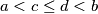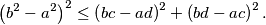### IMO Shortlist 1996 problem G6

Kvaliteta:
Avg: 0,0
Težina:
Avg: 8,0
Let the sides of two rectangles be$\{a,b\}$ and$\{c,d\},$ respectively, with$a < c \leq d < b$ and$ab < cd.$ Prove that the first rectangle can be placed within the second one if and only ifIzvor: Međunarodna matematička olimpijada, shortlist 1996